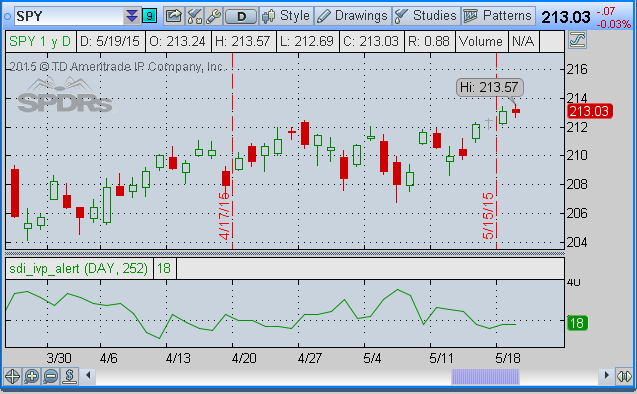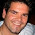## Tuesday, May 19, 2015

### thinkscript included: sdi_ivp_alert - implied volatility percentile that is compatible with study alerts.

it was brought to my attention that implied volatility percentile (aka rank) was not an available selection when composing alerts. this is because, as far as tos is concerned, iv percentile is a number that only has relevence as a stat on the trade tab or as a study filter on the scan tab. the fact that people might want to set alerts when iv percentile reaches a level of interest perhaps has eluded them?

into this void i bring the sdi_ivp_alert study. this chart study merely plots the iv percentile in a fashion that is compatible with alerts. here's how it looks on a chart:spy with sdi_ivp_alert study
just a simple line plot, no bells and whistles, those you have to add via marketWatch/alerts/study alert:

here's the code:

################################
# author: allen everhart
# date: 19may2015
# copylefts reserved. This is free software. That means you are free
# to use or modify it for your own usage but not for resale.
# Help me get the word out about my blog by keeping this header
# in place.

input period = AggregationPeriod.DAY ;
#hint period: time period to use for aggregating implied volatility. sdi_ivp_alert rev: 1.0.0 http://www.smallDogInvestor.com
input length =252 ;
def ivGapHi = if isnan(imp_volatility(period=period)) then double.POSITIVE_INFINITY else imp_volatility(period=period);
#hint: Displays the Implied Volatility Percent Range (aka rank, aka pecentile) as an alert-compatible plot. rev: 1.0.0 http://www.smallDogInvestor.com
def ivGapLo = if isnan(imp_volatility(period=period)) then double.NEGATIVE_INFINITY else imp_volatility(period=period);
def periodHigh = highest( ivGapLo,length=length);
# copylefts reserved. This is free software. That means you are free
# to use or modify it for your own usage but not for resale.
# Help me get the word out about my blog by keeping this header
# in place. source: smalldoginvestor.com
def periodLow = lowest( ivGapHi, length=length);
def ivRange = periodHigh - periodLow ;
plot ivp = round( 100*(imp_volatility(period=period) - periodLow)/ivRange, 0);

1.Just another great Script. Much appreciate. And you even provide Atom Feeds. Just subscribed. Thanks Ellen

2.This comment has been removed by the author.

3.This comment has been removed by the author.

4.Allen, I like your script. I've been using the standard IV_percentile function in TOS watchlists and scans to bring my attention to the underlyings with the fattest IV out there. However, even though it is labeled IV_Percentile in TOS, the script behind it is really just calculating IV_Rank (i.e. current IV relative to range high and low); much like the script you provide above.

From personal experience, and as confirmed from the Dr. Data Data Science show on TastyTrade.com, the IV_Rank does not represent the bulk of the distribution as well as the IV_Percentile does (i.e. current IV relative to the entire IV distribution). In fact, they can be vastly different from each other; enough such that scans based on the IV_Rank can miss many underlyings with fat premium that are also great trade candidates. The technical problem is that the thinkscript fold function is needed to calculate the IV_percentile; and although it is supported by the charts, it is not supported by the watchlists or scans.

However, I found a solution! I've coded what I call an IV_Factor that calculates the number of standard deviations (SD) the current IV is above the average IV, It can be used as a relative IV ranking scheme [(IV-AvgIV)/StDevIV)], just like the IV_Percentile. Since IV follows a normal distribution and is mean reverting, this statistic is pretty handy with 97.7% of the data less than 2 SD and 84.1% less than 1 SD. It works quite well ranking wise and ranks the underlyings in roughly the same order as the IV_percentile would within +/- 1-2% IV_percentile due to the inherent data smoothing differences between the way the two calculations are performed. Close enough I say for TastyTraders who want to find the richest IV around.

Simply scan for IV_Factor > 0 (i.e. greater than the average) and rank them according to how many standard deviations the IV is above the average IV. The higher the number, the richer the premium relative to the distribution over the last year; with numbers above 2 being exponentially more and more rare. I have included the scan script below and and I'm also submitting it to TastyTrade for consideration on their GameChangers show.

-------------------------------------------------------------------

#IV_Factor: Calculates the number of standard deviations above the average IV of the current IV value for the evaluated time period. Values greater than 0 represent higher than average IV relative to the number of standard deviations. 95% of all the IV should fall within 2 standard deviations since IV is normally distributed. High IV results in rich premium to sell. This acts as a surrogate for the true IV_percentile since the fold fuction necessary to calculate the IV percentile is not supported in watchlists or scan thinkscript.
#Created by Jonathon Bill Walker 01/09/16
# copyrights reserved. This is free software. That means you are free to use or modify it for your own usage but not for resale.

##################################
# INPUTS
##################################
declare lower;
input length = 13;
input TimePeriod = 252;
def vol = imp_volatility();
def data = if !IsNaN(vol) then vol else vol[-1];

##################################
# IV Factor
##################################
def AvgIV = average(vol,TimePeriod);
def SDIV = StDev(vol,TimePeriod);
plot IV_Factor = (vol - AvgIV) /(SDIV) ;

-------------------------------------------------------------------

5.It is also known as the Z-statistic, in this case for 1 tail only.